1. /
2. CBSE
3. /
4. Class 12
5. /
6. Physics
7. /
8. NCERT Solutions class 12...

# NCERT Solutions class 12 physics Nuclei Part 2### myCBSEguide App

Download the app to get CBSE Sample Papers 2023-24, NCERT Solutions (Revised), Most Important Questions, Previous Year Question Bank, Mock Tests, and Detailed Notes.

NCERT Solutions class 12 physics Nuclei Part 2 Class 12 Physics book solutions are available in PDF format for free download. These ncert book chapter wise questions and answers are very helpful for CBSE board exam. CBSE recommends NCERT books and most of the questions in CBSE exam are asked from NCERT text books. Class 12 Physics chapter wise NCERT solution for Physics part 1 and Physics part 2 for all the chapters can be downloaded from our website and myCBSEguide mobile app for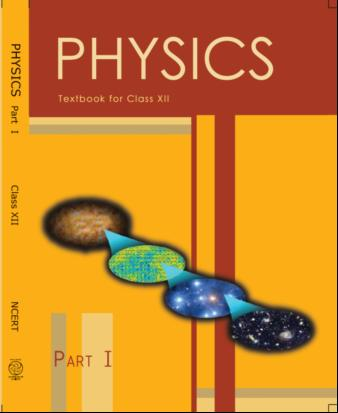## NCERT Class 12 Physics Chapter-wise Solutions

• 1 – Electric Charges and Fields
• 2 – Electrostatic Potential and Capacitance
• 3 – Current Electricity
• 4 – Moving Charges and Magnetism
• 5 – Magnetism and Matter
• 6 – Electromagnetic Induction
• 7 – Alternating Current
• 8 – Electromagnetic Waves
• 9 – Ray Optics and Optical Instruments
• 10 – Wave Optics
• 11 – Dual Nature of Radiation and Matter
• 12 – Atoms
• 13 – Nuclei
• 14 – Semiconductor Electronic: Material, Devices and Simple Circuits
• 15 – Communication Systems

## CHAPTER 13 NUCLEI

• 13.1 Introduction
• 13.2 Atomic Masses and Composition of Nucleus
• 13.3 Size of the Nucleus
• 13.5 Nuclear Force
• 13.7 Nuclear Energy

## NCERT Solutions class-12 physics Nuclei Part-2

16:  Suppose, we think of fission of a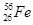nucleus into two equal fragments,. Is the fission energetically possible? Argue by working out Q of the process. Given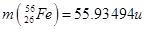and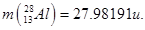.

17: The fission properties of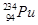are very similar to those of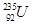.

The average energy released per fission is 180 MeV. How much energy, in MeV, is released if all the atoms in 1 kg of pure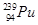undergo fission?

18:  A 1000 MW fission reactor consumes half of its fuel in 5.00 y. How muchdid it contain initially? Assume that the reactor operates 80% of the time, that all the energy generated arises from the fission ofand that this nuclide is consumed only by the fission process.

19:  How long can an electric lamp of 100W be kept glowing by fusion of 2.0 kg of deuterium? Take the fusion reaction as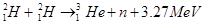20:  Calculate the height of the potential barrier for a head on collision of two deuterons. (Hint: The height of the potential barrier is given by the Coulomb repulsion between the two deuterons when they just touch each other. Assume that they can be taken as hard spheres of radius 2.0 fm.)

21:  From the relation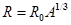, where R0is a constant and A is the mass number of a nucleus, show that the nuclear matter density is nearly constant (i.e. independent of A).

22:  For the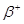(positron) emission from a nucleus, there is another competing process known as electron capture (electron from an inner orbit, say, the K – shell, is captured by the nucleus and a neutrino is emitted).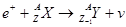Show that ifemission is energetically allowed, electron capture is necessarily allowed but not vice – versa.

23: In a periodic table the average atomic mass of magnesium is given as 24.312 u. The average value is based on their relative natural abundance on earth. The three isotopes and their masses are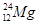(23.98504u),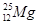(24.98584u) and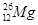(25.98259u). The natural abundance ofis 78.99% by mass. Calculate the abundances of other two isotopes.

24:  The neutron separation energy is defined as the energy required to remove a neutron from the nucleus. Obtain the neutron separation energies of the nuclei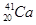and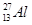from the following data: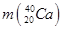= 39.962591 u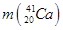= 40.962278 u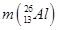= 25.986895 u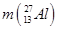= 26.981541 u

25:  A source contains two phosphorous radio nuclides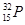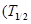=14.3d) and= 25.3d). Initially, 10% of the decays come from. How long one must wait until 90% do so?

26:  Under certain circumstances, a nucleus can decay by emitting a particle more massive than an α-particle. Consider the following decay processes: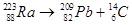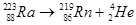Calculate the Q-values for these decays and determine that both are energetically allowed.

27:  Consider the fission of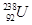by fast neutrons. In one fission event, no neutrons are emitted and the final end products, after the beta decay of the primary fragments, are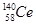and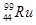. Calculate Q for this fission process.

The relevant atomic and particle masses are

m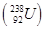=238.05079 u

m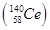=139.90543 u

m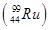= 98.90594 u

### 28:  Consider the D – T reaction (deuterium – tritium fusion)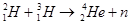(a) Calculate the energy released in MeV in this reaction from the data: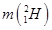= 2.014102 u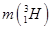= 3.016049 u

(b)Consider the radius of both deuterium and tritium to be approximately 2.0 fm. What is the kinetic energy needed to overcome the coulomb repulsion between the two nuclei? To what temperature must the gas be heated to initiate the reaction? (Hint: Kinetic energy required for one fusion event =average thermal kinetic energy available with the interacting particles = 2(3kT/2); k = Boltzman’s constant, T = absolute temperature.)

29:  Calculate and compare the energy released by a) fusion of 1.0 kg of hydrogen deep within Sun and b) the fission of 1.0 kg of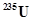in a fission reactor.

30:  Suppose India had a target of producing by 2020 AD, 200,000 MW of electric power, ten percent of which was to be obtained from nuclear power plants. Suppose we are given that, on an average, the efficiency of utilization (i.e. conversion to electric energy) of thermal energy produced in a reactor was 25%. How much amount of fissionable uranium would our country need per year by 2020? Take the heat energy per fission ofto be about 200MeV.

## NCERT Solutions for Class 12 Physics